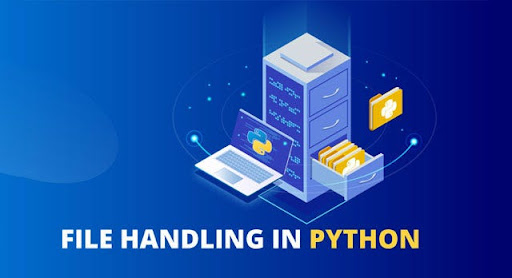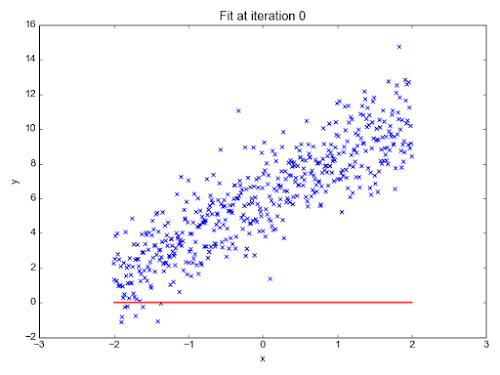## Posts

Showing posts from July, 2020

### File Handling with Python || Python programming tutorialIn File Handling,  we will be working on a file in our computer and doing various operations on it. Check out my previous blog on Python programming if you want to learn from scratch. Let us say we have a file name poem.txt and we need to perform various operations on that file. Reading the whole file and printing it First, we need to open that text file in our python program using a function  open(). The  open()  function will take two arguments filename and set our intentions. Now, you must be wondering about the second argument i.e. we need to specify our purpose of using that file  Read, Write  and  Append Above all operations can be specified by with different alphabets: Read - 'r' Write - 'w' Append - 'a' Now we will implement programs with this knowledge Program -  f = open("poem.txt", "r") f.read() Counting Number of Lines in our file We will divide this process into various steps : To read a single line of a file we can use readlin

### Getting Started with your first Machine Learning Algorithm - Linear Regression || First step towards MLLinear Regression is a Supervised Learning Algorithm. This is probably the first algorithm taught when you start learning ML. Now as a beginner you must be wondering "What is a Supervised Learning??". So machine learning can be divided mainly into 3 types: Supervised Learning Unsupervised Learning Reinforcement Learning Supervised Learning is a technique in which we use a labelled dataset to train a model. In short, you can say there is a supervisor who will tell whether the predictions model is making is correct or not. This supervisor decides about based on the labels provided in the datasets. Labels are the correct values for a particular training example. We will deal later with the other two techniques of ML. In supervised learning, we can classify problems into 2 different categories: Regression - problems in which we need to predict continuous value as an output. Classification - where we need to classify the training examples in a particular category. We plot all t